Select Page

# CBSE Maths 12 Science MCQ Integrals Answers in English

CBSE Maths 12 Science MCQ Integrals Answers in English to enable students to get Answers in a narrative video format for the specific question.

Expert Teacher provides CBSE Maths 12 Science MCQ Integrals Answers through Video Answers in English language. This video solution will be useful for students to understand how to write an answer in exam in order to score more marks. This teacher uses a narrative style for a question from Integrals not only to explain the proper method of answering question, but deriving right answer too.

Please find the question below and view the Answer in a narrative video format.

Question:

## Similar Questions from CBSE, 12th Science, Maths, Integrals

Question 1 :  Find the integral of the function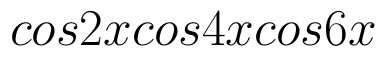. (View Answer Video)

Question 2 : Evaluate :(View Answer Video)

Question 3 : Evaluate :(View Answer Video)

Question 4 : Evaluate:as limit of sums.  (View Answer Video)

Question 5 : Evaluate :. (View Answer Video)

### Probability

Question 1 : If P(A)=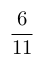, P(B)=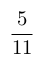and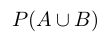=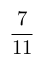, find. (View Answer Video)

Question 2 :  A coin is tossed three times. Find P(F/E), where E : at most two tails and F : atleast one tail.  (View Answer Video)

Question 3 : If E and F are two events such that,, Find P(E or F).         (View Answer Video)

Question 4 : A speaks truth in 60% of the cases, while B in 90% of cases. In what percent of cases are they likely to contradict each other in stating the same fact? In the cases of contradiction do you think, the statement B will carry more weight as he speaks truth in more number of cases than A?    (View Answer Video)

Question 5 : A problem in mathematics is given to 4 students A, B, C, D. Their chances of solving the problem, respectively, areand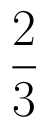. What is the probability that atmost one of them solve the problem?  (View Answer Video)

### Determinant

Question 1 : If the area of a triangle is 35 sq units with vertices (2, -6), (5, 4) and (k, 4). Then k is: (View Answer Video)

Question 3 : Find the equation of the line joining (1, 2) and (3, 6) using determinants. (View Answer Video)

Question 5 : Let, where. THhen (View Answer Video)

### Differential Equations

Question 1 : Write the degree of the differential equation(View Answer Video)

Question 2 : Show that the solution of differential equation :(View Answer Video)

Question 3 : Write the differential equation formed from the equation y = mx + c, where m and c are arbitrary constants.when x = 1,(View Answer Video)
Question 5 : Find the particular solution of the differential equationgiven that y = 1 when x = 0.  (View Answer Video)